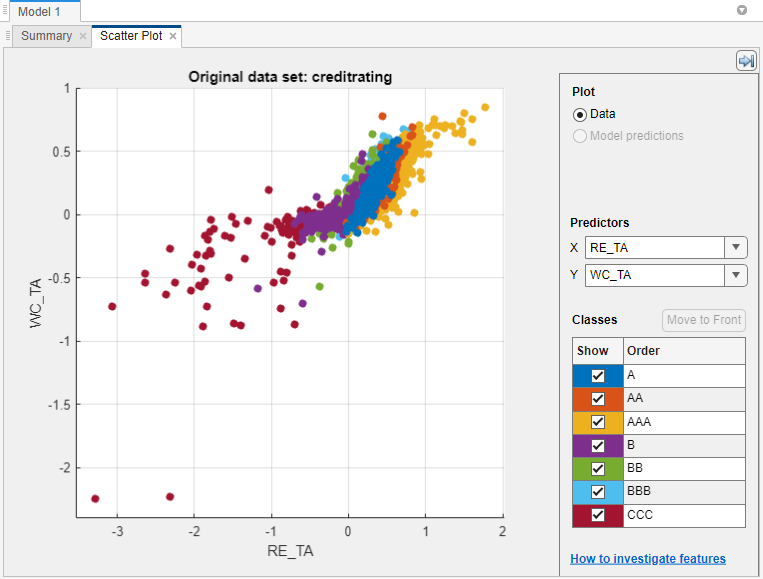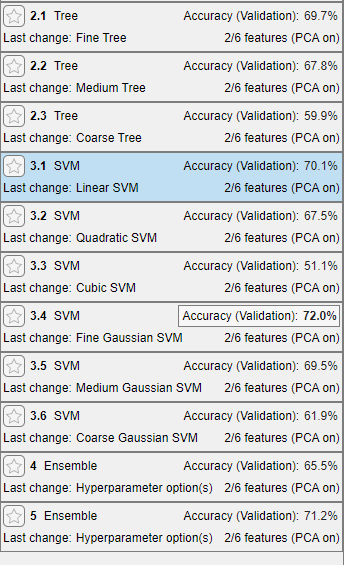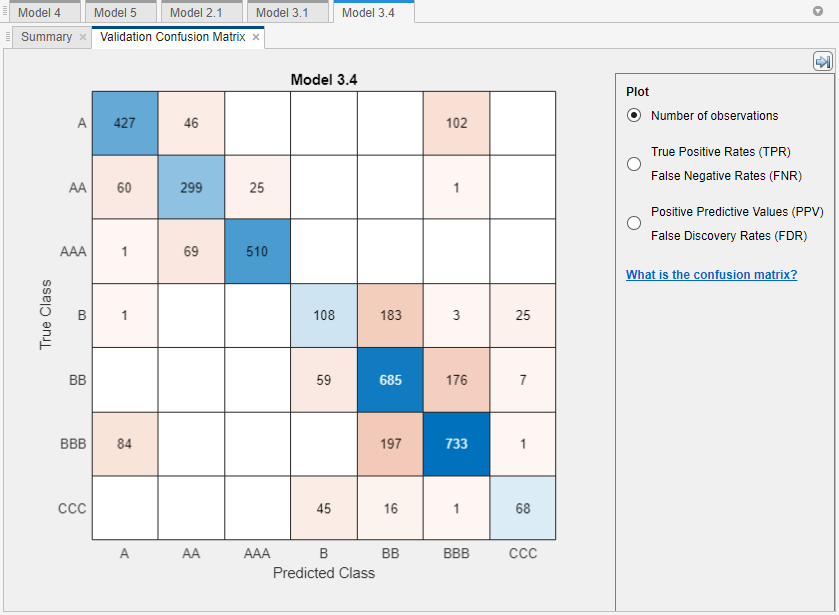## Code Generation and Classification Learner App

Classification Learner is well suited for choosing and training classification models interactively, but it does not generate C/C++ code that labels data based on a trained model. The button in the Export section of the Classification Learner app generates MATLAB code for training a model but does not generate C/C++ code. This example shows how to generate C code from a function that predicts labels using an exported classification model. The example builds a model that predicts the credit rating of a business given various financial ratios, according to these steps:

1. Use the credit rating data set in the file `CreditRating_Historical.dat`, which is included with Statistics and Machine Learning Toolbox™.

2. Reduce the data dimensionality using principal component analysis (PCA).

3. Train a set of models that support code generation for label prediction.

4. Export the model with the minimum 5-fold, cross-validated classification accuracy.

5. Generate C code from an entry-point function that transforms the new predictor data and then predicts corresponding labels using the exported model.

Load sample data and import the data into the Classification Learner app. Review the data using scatter plots and remove unnecessary predictors.

Use `readtable` to load the historical credit rating data set in the file `CreditRating_Historical.dat` into a table.

```creditrating = readtable('CreditRating_Historical.dat'); ```

On the Apps tab, click Classification Learner.

In Classification Learner, on the Classification Learner tab, in the File section, click New Session and select From Workspace.

In the New Session from Workspace dialog box, select the table `creditrating`. All variables, except the one identified as the response, are double-precision numeric vectors. Click to compare classification models based on the 5-fold, cross-validated classification accuracy.

Classification Learner loads the data and plots a scatter plot of the variables `WC_TA` versus `ID`. Because identification numbers are not helpful to display in a plot, choose `RE_TA` for X under Predictors.The scatter plot suggests that the two variables can separate the classes `AAA`, `BBB`, `BB`, and `CCC` fairly well. However, the observations corresponding to the remaining classes are mixed into these classes.

Identification numbers are not helpful for prediction. Therefore, in the Features section, click . In the Feature Selection dialog box, clear the ID check box, and click . You can also remove unnecessary predictors from the beginning by using the check boxes in the New Session from Workspace dialog box. This example shows how to remove unused predictors for code generation when you have included all predictors.

### Enable PCA

Enable PCA to reduce the data dimensionality.

In the section, click . In the Advanced PCA Options dialog box, select Enable PCA, and click . This action applies PCA to the predictor data, and then transforms the data before training the models. Classification Learner uses only components that collectively explain 95% of the variability.

### Train Models

Train a set of models that support code generation for label prediction. For a list of models in Classification Learner that support code generation, see Generate C Code for Prediction.

Select the following classification models and options, which support code generation for label prediction, and then perform cross-validation (for more details, see Introduction to Code Generation). To select each model, in the Model Type section, click the Show more arrow, and then click the model. After selecting a model and specifying any options, click in the Training section.

Models and Options to SelectDescription
Under Decision Trees, select All TreesClassification trees of various complexities
Under Support Vector Machines, select All SVMsSVMs of various complexities and using various kernels. Complex SVMs require time to fit.
Under Ensemble Classifiers, select Boosted Trees. In the Model Type section, click . In the dialog box, reduce Maximum number of splits to 5 and increase Number of learners to 100. Click .Boosted ensemble of classification trees
Under Ensemble Classifiers, select Bagged Trees. In the Model Type section, click . In the dialog box, increase Maximum number of splits to 50 and increase Number of learners to 100. Click .Random forest of classification trees

After cross-validating each model type, the Models pane displays each model and its 5-fold, cross-validated classification accuracy, and highlights the model with the best accuracy.Select the model that yields the maximum 5-fold, cross-validated classification accuracy, which is the error-correcting output codes (ECOC) model of Fine Gaussian SVM learners. With PCA enabled, Classification Learner uses two predictors out of six.

In the Plots section, click the arrow to open the gallery, and then click in the Validation Results group.The model does well distinguishing between `A`, `B`, and `C` classes. However, the model does not do as well distinguishing between particular levels within those groups, the lower B levels in particular.

### Export Model to Workspace

Export the model to the MATLAB® Workspace and save the model using `saveLearnerForCoder`.

In the Export section, click , and then select Export Compact Model. Click in the dialog box.

The structure `trainedModel` appears in the MATLAB Workspace. The field `ClassificationSVM` of `trainedModel` contains the compact model.

At the command line, save the compact model to a file called `ClassificationLearnerModel.mat` in your current folder.

`saveLearnerForCoder(trainedModel.ClassificationSVM,'ClassificationLearnerModel')`

### Generate C Code for Prediction

Prediction using the object functions requires a trained model object, but the `-args` option of `codegen` (MATLAB Coder) does not accept such objects. Work around this limitation by using `saveLearnerForCoder` and `loadLearnerForCoder`. Save a trained model by using `saveLearnerForCoder`. Then, define an entry-point function that loads the saved model by using `loadLearnerForCoder` and calls the `predict` function. Finally, use `codegen` to generate code for the entry-point function.

#### Preprocess Data

Preprocess new data in the same way you preprocess the training data.

To preprocess, you need the following three model parameters:

• `removeVars` — Column vector of at most `p` elements identifying indices of variables to remove from the data, where `p` is the number of predictor variables in the raw data

• `pcaCenters` — Row vector of exactly `q` PCA centers

• `pcaCoefficients``q`-by-`r` matrix of PCA coefficients, where `r` is at most `q`

Specify the indices of predictor variables that you removed while selecting data using in Classification Learner. Extract the PCA statistics from `trainedModel`.

```removeVars = 1; pcaCenters = trainedModel.PCACenters; pcaCoefficients = trainedModel.PCACoefficients; ```

Save the model parameters to a file named `ModelParameters.mat` in your current folder.

`save('ModelParameters.mat','removeVars','pcaCenters','pcaCoefficients');`

#### Define Entry-Point Function

An entry-point function is a function you define for code generation. Because you cannot call any function at the top level using `codegen`, you must define an entry-point function that calls code-generation-enabled functions, and then generate C/C++ code for the entry-point function by using `codegen`.

In your current folder, define a function named `mypredictCL.m` that:

• Accepts a numeric matrix (`X`) of raw observations containing the same predictor variables as the ones passed into Classification Learner

• Loads the classification model in `ClassificationLearnerModel.mat` and the model parameters in `ModelParameters.mat`

• Removes the predictor variables corresponding to the indices in `removeVars`

• Transforms the remaining predictor data using the PCA centers (`pcaCenters`) and coefficients (`pcaCoefficients`) estimated by Classification Learner

• Returns predicted labels using the model

```function label = mypredictCL(X) %#codegen %MYPREDICTCL Classify credit rating using model exported from %Classification Learner % MYPREDICTCL loads trained classification model (SVM) and model % parameters (removeVars, pcaCenters, and pcaCoefficients), removes the % columns of the raw matrix of predictor data in X corresponding to the % indices in removeVars, transforms the resulting matrix using the PCA % centers in pcaCenters and PCA coefficients in pcaCoefficients, and then % uses the transformed data to classify credit ratings. X is a numeric % matrix with n rows and 7 columns. label is an n-by-1 cell array of % predicted labels. % Load trained classification model and model parameters SVM = loadLearnerForCoder('ClassificationLearnerModel'); data = coder.load('ModelParameters'); removeVars = data.removeVars; pcaCenters = data.pcaCenters; pcaCoefficients = data.pcaCoefficients; % Remove unused predictor variables keepvars = 1:size(X,2); idx = ~ismember(keepvars,removeVars); keepvars = keepvars(idx); XwoID = X(:,keepvars); % Transform predictors via PCA Xpca = bsxfun(@minus,XwoID,pcaCenters)*pcaCoefficients; % Generate label from SVM label = predict(SVM,Xpca); end ```

#### Generate Code

Because C and C++ are statically typed languages, you must determine the properties of all variables in the entry-point function at compile time. Specify variable-size arguments using `coder.typeof` (MATLAB Coder) and generate code using the arguments.

Create a double-precision matrix called `x` for code generation using `coder.typeof` (MATLAB Coder). Specify that the number of rows of `x` is arbitrary, but that `x` must have `p` columns.

```p = size(creditrating,2) - 1; x = coder.typeof(0,[Inf,p],[1 0]);```

For more details about specifying variable-size arguments, see Specify Variable-Size Arguments for Code Generation.

Generate a MEX function from `mypredictCL.m`. Use the `-args` option to specify `x` as an argument.

`codegen mypredictCL -args x`

`codegen` generates the MEX file `mypredictCL_mex.mexw64` in your current folder. The file extension depends on your platform.

#### Verify Generated Code

Verify that the MEX function returns the expected labels.

Remove the response variable from the original data set, and then randomly draw 15 observations.

```rng('default'); % For reproducibility m = 15; testsampleT = datasample(creditrating(:,1:(end - 1)),m);```

Predict corresponding labels by using `predictFcn` in the classification model trained by Classification Learner.

`testLabels = trainedModel.predictFcn(testsampleT);`

Convert the resulting table to a matrix.

`testsample = table2array(testsampleT);`

The columns of `testsample` correspond to the columns of the predictor data loaded by Classification Learner.

Pass the test data to `mypredictCL`. The function `mypredictCL` predicts corresponding labels by using `predict` and the classification model trained by Classification Learner.

`testLabelsPredict = mypredictCL(testsample);`

Predict corresponding labels by using the generated MEX function `mypredictCL_mex`.

`testLabelsMEX = mypredictCL_mex(testsample);`

Compare the sets of predictions.

`isequal(testLabels,testLabelsMEX,testLabelsPredict)`
```ans = logical 1```

`isequal` returns logical 1 (true) if all the inputs are equal. `predictFcn`, `mypredictCL`, and the MEX function return the same values.